CF1080C Masha and two friends

• 122通过
• 186提交
• 题目来源
• 评测方式 RemoteJudge
• 标签
• 难度 省选/NOI-
• 时空限制 1000ms / 256MB
• 提示：收藏到任务计划后，可在首页查看。

题目描述

Masha 有一个宽度为 $n$，长度为 $m$ 的国际象棋盘。

这个象棋盘左下角的坐标为 $(1,1)$，且颜色为白色。(象棋盘是黑白相间的)

现在 Maxim 首先选择了一个矩形，并把这个矩形内的象棋盘刷成了黑色，然后 Denis 又选择了一个矩形并把这个矩形内的象棋盘刷成了白色。 (注意先后顺序)

请输出这个象棋盘最后有多少个黑色格子，多少个白色格子。

输入格式

第一行一个整数 $t$，表示有 $t$ 组数据。

每组数据第一行两个整数 $n,m$

接下来一行四个整数 $x1,y1,x2,y2$，表示 Maxim 选定矩形的左下角坐标和右上角坐标。

接下来一行也是四个整数 $x3,y3,x4,y4$，表示 Denix 选定矩形的左下角坐标和右上角坐标。

输出格式

对于每一组数据，输出一行两个整数，表示白色格子的数量和黑色格子的数量 (白前黑后)

数据范围

$1\leq t\leq 10^3$

$1 \leq n,m \leq 10^9$

$1 \leq x1 \leq x2 \leq m$, $1 \leq x3 \leq x4 \leq m$

$1 \leq y1 \leq y2 \leq n$, $1 \leq y3 \leq y4 \leq n$

题目描述

Recently, Masha was presented with a chessboard with a height of $n$ and a width of $m$ .

The rows on the chessboard are numbered from $1$ to $n$ from bottom to top. The columns are numbered from $1$ to $m$ from left to right. Therefore, each cell can be specified with the coordinates $(x,y)$ , where $x$ is the column number, and $y$ is the row number (do not mix up).

Let us call a rectangle with coordinates $(a,b,c,d)$ a rectangle lower left point of which has coordinates $(a,b)$ , and the upper right one — $(c,d)$ .

The chessboard is painted black and white as follows: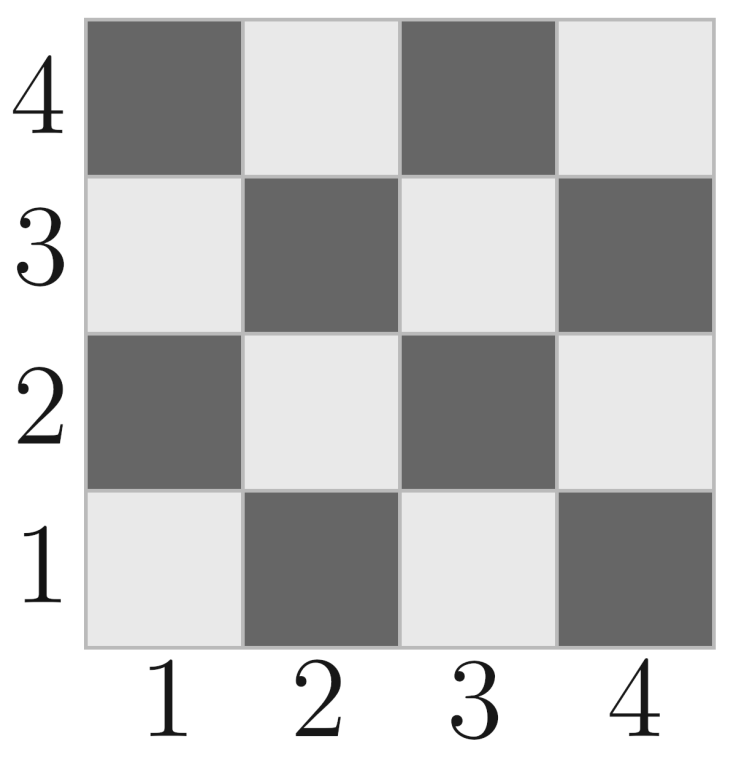An example of a chessboard.Masha was very happy with the gift and, therefore, invited her friends Maxim and Denis to show off. The guys decided to make her a treat — they bought her a can of white and a can of black paint, so that if the old board deteriorates, it can be repainted. When they came to Masha, something unpleasant happened: first, Maxim went over the threshold and spilled white paint on the rectangle $(x_1,y_1,x_2,y_2)$ . Then after him Denis spilled black paint on the rectangle $(x_3,y_3,x_4,y_4)$ .

To spill paint of color $color$ onto a certain rectangle means that all the cells that belong to the given rectangle become $color$ . The cell dyeing is superimposed on each other (if at first some cell is spilled with white paint and then with black one, then its color will be black).

Masha was shocked! She drove away from the guests and decided to find out how spoiled the gift was. For this, she needs to know the number of cells of white and black color. Help her find these numbers!

输入输出格式

输入格式：

The first line contains a single integer $t$ ( $1 \le t \le 10^3$ ) — the number of test cases.

Each of them is described in the following format:

The first line contains two integers $n$ and $m$ ( $1 \le n,m \le 10^9$ ) — the size of the board.

The second line contains four integers $x_1$ , $y_1$ , $x_2$ , $y_2$ ( $1 \le x_1 \le x_2 \le m, 1 \le y_1 \le y_2 \le n$ ) — the coordinates of the rectangle, the white paint was spilled on.

The third line contains four integers $x_3$ , $y_3$ , $x_4$ , $y_4$ ( $1 \le x_3 \le x_4 \le m, 1 \le y_3 \le y_4 \le n$ ) — the coordinates of the rectangle, the black paint was spilled on.

输出格式：

Output $t$ lines, each of which contains two numbers — the number of white and black cells after spilling paint, respectively.

输入输出样例

输入样例#1： 复制
5
2 2
1 1 2 2
1 1 2 2
3 4
2 2 3 2
3 1 4 3
1 5
1 1 5 1
3 1 5 1
4 4
1 1 4 2
1 3 4 4
3 4
1 2 4 2
2 1 3 3

输出样例#1： 复制
0 4
3 9
2 3
8 8
4 8


说明

Explanation for examples:

The first picture of each illustration shows how the field looked before the dyes were spilled. The second picture of each illustration shows how the field looked after Maxim spoiled white dye (the rectangle on which the dye was spilled is highlighted with red). The third picture in each illustration shows how the field looked after Denis spoiled black dye (the rectangle on which the dye was spilled is highlighted with red).

In the first test, the paint on the field changed as follows: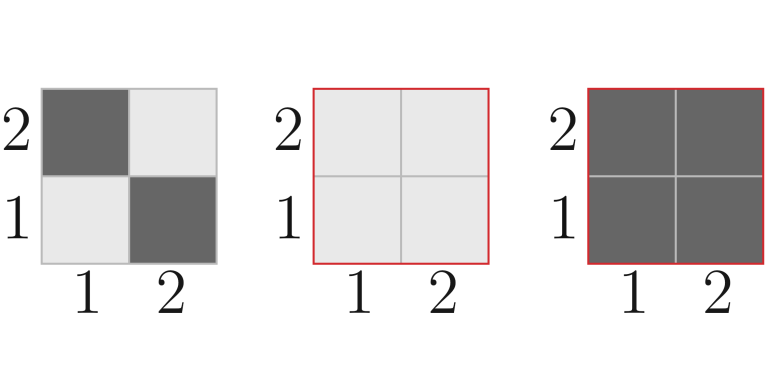In the second test, the paint on the field changed as follows: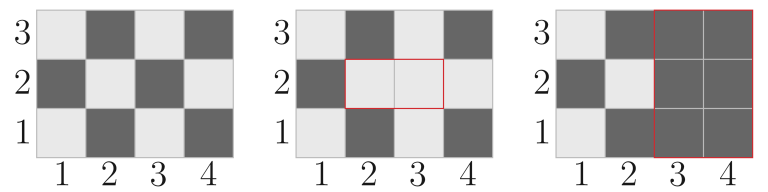In the third test, the paint on the field changed as follows: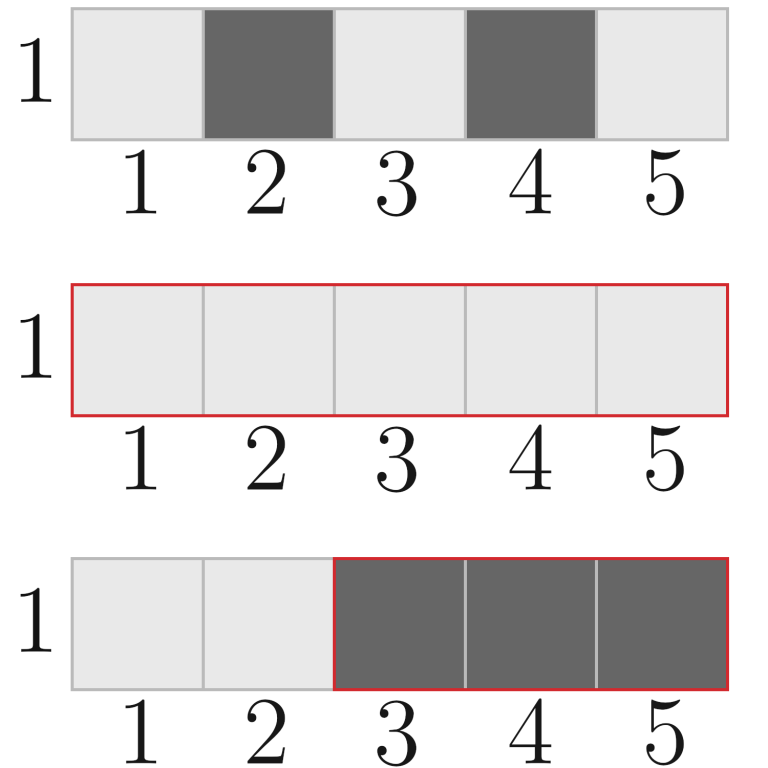In the fourth test, the paint on the field changed as follows:In the fifth test, the paint on the field changed as follows: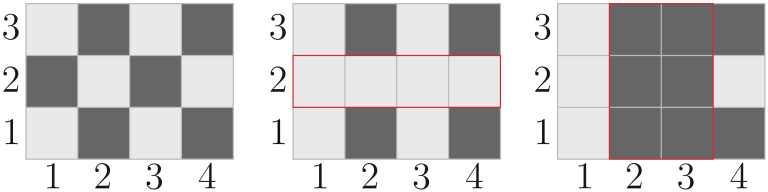提示
标程仅供做题后或实在无思路时参考。
请自觉、自律地使用该功能并请对自己的学习负责。
如果发现恶意抄袭标程，将按照I类违反进行处理。